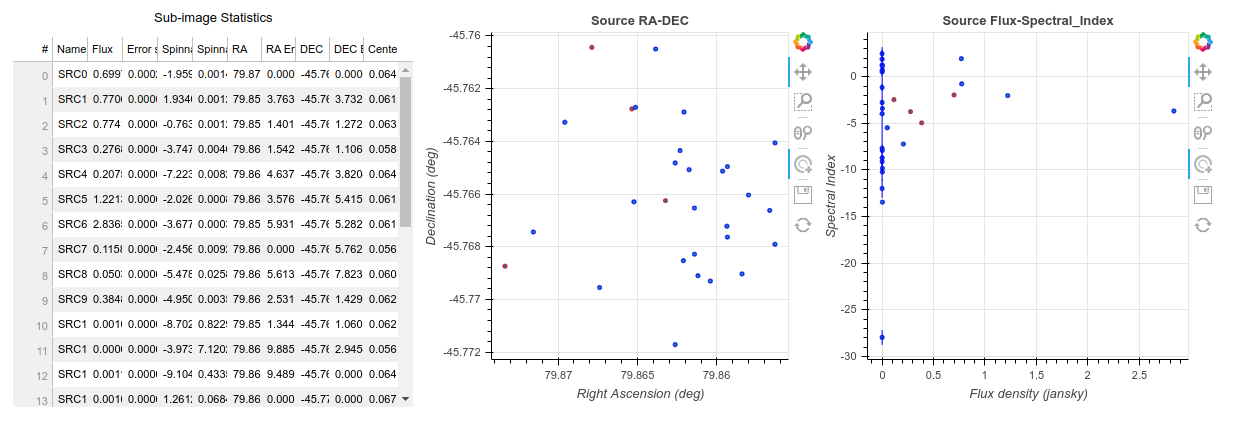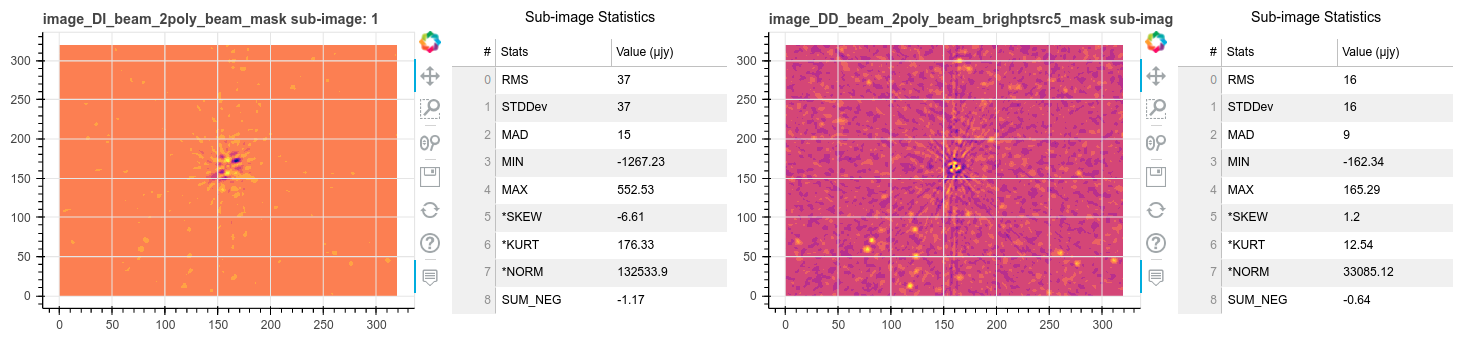# aimfast#

An Astronomical Image Fidelity Assessment Tool

# Introduction#

Image fidelity is a measure of the accuracy of the reconstructed sky brightness distribution. A related metric, dynamic range, is a measure of the degree to which imaging artifacts around strong sources are suppressed, which in turn implies a higher fidelity of the on-source reconstruction. Moreover, the choice of image reconstruction algorithm and source finder also affects the estimate on-source brightness distribution.

# Fidelity Matrices#

## Image dynamic range#

Dynamic range is a measure of the degree to which imaging artifacts around strong sources are suppressed, which in turn implies a higher fidelity of the on-source reconstruction. Here we determine it in three ways: Obtaining the quotient of - highest peak flux ($$flux_{peak}$$) and the absolute of the minimum flux ($$flux_{min}$$) around the peak in the residual image. - highest peak flux ($$flux_{peak}$$) and the rms flux ($$flux_{local_rms}$$) around the peak in the residual image. - highest peak flux ($$flux_{peak}$$) and the rms flux ($$flux_{grobal_rms}$$) in the residual image.

(1)#$DR = \frac{flux_{peak}}{\left | {flux_{min}} \right | } (1) DR = \frac{flux_{peak}}{\left | {flux_{local_rms}} \right | } (2) DR = \frac{flux_{peak}}{\left | {flux_{global_rms}} \right | } (3)$

## Statistical moments of distribution#

The mean and the variance provide information on the location (general value of the residual flux) and variability (spread, dispersion) of a set of numbers, and by doing so, provide some information on the appearance of the distribution of residual flux in the residual image. The mean and variance are calculated as follows respectively

(2)#$MEAN = \frac{1}{n}\sum_{i=1}^{n}(x_{i}) (4)$

and

(3)#$VARIANCE = \frac{1}{n}\sum_{i=1}^{n}(x_{i} - \overline{x})^2 (5)$

whereby

(4)#$STD\_DEV = \sqrt{VARIANCE} (6)$

The third and fourth moments are the skewness and kurtosis respectively. The skewness is the measure of the symmetry of the shape and kurtosis is a measure of the flatness or peakness of a distribution. This moments are used to characterize the residual flux after performing calibration and imaging, therefore for ungrouped data, the r-th moment is calculated as follows:

(5)#$m_r = \frac{1}{n}\sum_{i=1}^{n}(x_i - \overline{x})^r (7)$

The coefficient of skewness, the 3-rd moment, is obtained by

(6)#$SKEWNESS = \frac{m_3}{{m_2}^{\frac{3}{2}}} (8)$

If there is a long tail in the positive direction, skewness will be positive, while if there is a long tail in the negative direction, skewness will be negative.

The coefficient kurtosis, the 4-th moment, is obtained by

(7)#$KURTOSIS = \frac{m_4}{{m_2}^{2}} (9)$

Smaller values (in magnitude) indicate a flatter, more uniform distribution.

Furthermore, there is median absolute deviation which is a measure of how distributed is the residual data with regards to the median. This can be compared with the standard deviation to verify that the residuals are noise-like (and Gaussian).

# Installation#

Installation from source, working directory where source is checked out

$pip install .  This package is available on PYPI, allowing $ pip install aimfast


## Command line usage#

Get the statistics of the residual image

$aimfast --residual-image cube.residual.fits  Get the residual image stats and dynamic range in one step where argument -af is a factor to multiply the beam area to get target peak area: $ aimfast --residual-image cube.residual.fits --restored-image cube.image.fits -af 5


or using sky model file (e.g. tigger lsm.html):

$aimfast --residual-image cube.residual.fits --tigger-model model.lsm.html -af 5  NB: Outputs will be printed on the terminal and dumped into fidelity_results.json file. Moreover if the source file names are distinct the output results will be appended to the same json file. $ cat fidelity_results.json
${"cube.residual.fits": {"SKEW": 0.124, "KURT": 3.825, "STDDev": 5.5e-05, "MEAN": 4.747e-07, "MAD": 5e-05, "RMS": 5.5e-05}, "cube.image.fits": {"DR": 35.39, "deepest_negative": 10.48, "local_rms": 30.09, "global_rms": 35.39}}  Additionally, normality testing of the residual image can be performed using the D’Agostino (normaltest) and Shapiro-Wilk (shapiro) analysis, which returns a tuple result, e.g {‘NORM’: (123.3, 0.1)}, with the z-score and p-value respectively. $ aimfast --residual-image cube.residual.fits --normality-model normaltest


Many astronomical catalogues consist of numerous columns of source information, and often times there is an existing connection between corresponding pairs. aimfast allows visualising these relationships by generating interactive plots that can be easily distributed and only requires a modern web browser. A handful number of catalogue formats are supported; currently, this includes FITS, LSM and ASCII.

$aimfast -catalog PictorA_1-MFS-IQUV.image.pybdsm.lsm.html -x I -y spi -x-err I_err -y-err spi_err -x-label 'Flux Density (Jy)' -y-label 'SPI' -title 'Catalog Flux vs Spectral Index' -title-size 30pt -x-size 26pt -y-size 26pt -x-maj-size 18pt -y-maj-size 18pt --html-prefix pica-hot-flux$ aimfast -catalog PictorA_1-MFS-IQUV.image.pybdsm.lsm.html -x ra -y dec -x-err ra_err -y-err dec_err -x-label 'Right Ascension (deg)' -y-label 'Declination (deg)' -title 'Catalog RA vs DEC' -title-size 30pt -x-size 26pt -y-size 26pt -x-maj-size 18pt -y-maj-size 18pt  --html-prefix pica-hot-pos


The results are as follows:Figure 3: Example of 2D plots showing source properties from a catalogue. Left: Source RA vs Dec. Right Source flux density vs Spectral Index}#

Furthermore, a comparison of residual images can be performed as follows: To get random residual flux measurements in residual1.fits and residual2.fits images

$aimfast --compare-residuals residual1.fits residual2.fits --area-factor 2 -dp 1590 --html-prefix cluster1 --units milli -x-size 34pt -y-size 34pt -title-size 36pt -legend-size 25pt -x-maj-size 24pt -y-maj-size 24pt -units micro  where –area-factor is the number to multiply the beam size to get area and -dp is the number of data points to sample. In case the beam information is missing from the image header use –psf-image | -psf, the point spread function file or psf size in arcsec, otherwise a default of 5 arcsec will be used. To get the on source residual flux measurements in a residual1.fits and residual2.fits images $ aimfast --compare-residuals  residual1.fits residual2.fits -catalog model.lsm.html  --html-prefix cluster1 --units milli -x-size 34pt -y-size 34pt -title-size 36pt -legend-size 25pt -x-maj-size 24pt -y-maj-size 24pt -units micro


where -catalog is the catalog file name with source detections to locate exact source residuals. For random or on source residual noise comparisons, the plot on the left shows the residuals on image 1 and image 2 overlayed and the plot on the right shows the ratios. The colorbar shows the distance of the sources from the phase centre.

Figure 4. The random/source residual-to-residual ratio measurements

A comparison of residual images can also be performed by generating random locations on the image and calculating the statics, and overlying the results to distinguish the two images in question.

$aimfast --compare-residual-subimages residual1.fits residual2.fits -cps 1004,4960,320 --html-prefix cluster --units milli -x-size 15pt -y-size 15pt -bar-size 15pt  Generated sub-images with corresposnding statistics:Figure 5: A comparison of (320x320 Pixels) images of corrected residual data. Left: Direction-Independent corrections. Right: Direction-Dependent corrections.}# Moreover aimfast allows you to swiftly compare two (input-output) model catalogs. Currently source flux density and astrometry are examined. It returns an interactive html correlation plots, from which a .png file can be easily downloaded. $ aimfast --compare-models model1.lsm.html model2.lsm.html -tol 5


where -tol is the tolerance to cross-match sources in arcsec. Moreover -as flag can be used to compare all source irrespective of shape (otherwise only point-like source with maj<2” are used). Access to (sumss, nvss,) online catalogs is also provided, to allow comparison of local catalogs to remote catalogs.

$aimfast --compare-online model1.lsm.html --online-catalog nvss -tol 5  In the case where fits images are compared, aimfast can pre-install source finder of choice (pybdsf, aegean,) to generate a catalogs which are in turn compared: $ aimfast --compare-images image1.fits image1.fits --source-finder pybdsf -tol 5


After the first run attempt one of the outputs is source_finder.yml file, which provide all the possible parameters of the source finders. Otherwise this file can be generated and edited prior to the comparison:

$aimfast source-finder -gc my-source-finder.yml$ aimfast --compare-images image1.fits image2.fits --html-prefix cluster --units milli -x-size 16pt -y-size 16pt -title-size 28pt -legend-size 16pt -x-maj-size 16pt -y-maj-size 16pt -bar-size 16pt -bar-major-size 14pt -units micro


For Flux density, the more the data points rest on the y=x (or I_out=I_in), the more correlated the two models are.

$aimfast source-finder -gc my-source-finder.yml$ aimfast source-finder -c my-source-finder.yml -sf pybdsf